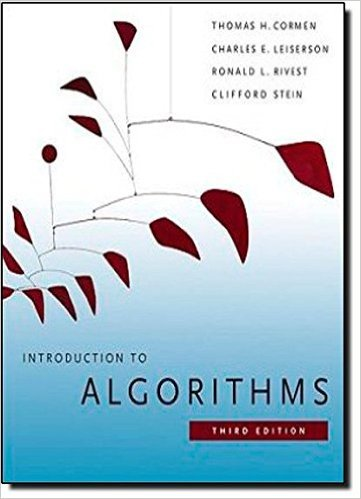×
Get Full Access to Introduction To Algorithms - 3 Edition - Chapter 2 - Problem 2-3
Get Full Access to Introduction To Algorithms - 3 Edition - Chapter 2 - Problem 2-3

×

# Correctness of Horners rule The following code fragmentISBN: 9780262033848 130

## Solution for problem 2-3 Chapter 2

Introduction to Algorithms | 3rd Edition

• Textbook Solutions
• 2901 Step-by-step solutions solved by professors and subject experts
• Get 24/7 help from StudySoup virtual teaching assistantsIntroduction to Algorithms | 3rd Edition

4 5 1 366 Reviews
30
5
Problem 2-3

Correctness of Horners rule The following code fragment implements Horners rule for evaluating a polynomial P .x/ D Xn kD0 akxk D a0 C x.a1 C x.a2 CC x.an1 C xan/// ; given the coefficients a0; a1;:::;an and a value for x: 1 y D 0 2 for i D n downto 0 3 y D ai C x y a. In terms of -notation, what is the running time of this code fragment for Horners rule? b. Write pseudocode to implement the naive polynomial-evaluation algorithm that computes each term of the polynomial from scratch. What is the running time of this algorithm? How does it compare to Horners rule? c. Consider the following loop invariant: At the start of each iteration of the for loop of lines 23, y D n X .iC1/ kD0 akCiC1xk : Interpret a summation with no terms as equaling 0. Following the structure of the loop invariant proof presented in this chapter, use this loop invariant to show that, at termination, y D Pn kD0 akxk. d. Conclude by arguing that the given code fragment correctly evaluates a polynomial characterized by the coefficients a0; a1;:::;an. 2-4 Inv

Step-by-Step Solution:
Step 1 of 3

Wednesday, August 30, 2017 12:59 PM Exam 1 Page 1 Exam 1 Page 2 2.3 Techniques for Computing Limits Wednesday, August 30, 20171:19 PM Exam 1 Page 1 Exam 1 Page 2 Exam 1 Page 3

Step 2 of 3

Step 3 of 3

##### ISBN: 9780262033848

Since the solution to 2-3 from 2 chapter was answered, more than 348 students have viewed the full step-by-step answer. The full step-by-step solution to problem: 2-3 from chapter: 2 was answered by , our top Engineering and Tech solution expert on 11/10/17, 05:55PM. This full solution covers the following key subjects: polynomial, horners, rule, Loop, invariant. This expansive textbook survival guide covers 35 chapters, and 151 solutions. This textbook survival guide was created for the textbook: Introduction to Algorithms, edition: 3. The answer to “Correctness of Horners rule The following code fragment implements Horners rule for evaluating a polynomial P .x/ D Xn kD0 akxk D a0 C x.a1 C x.a2 CC x.an1 C xan/// ; given the coefficients a0; a1;:::;an and a value for x: 1 y D 0 2 for i D n downto 0 3 y D ai C x y a. In terms of -notation, what is the running time of this code fragment for Horners rule? b. Write pseudocode to implement the naive polynomial-evaluation algorithm that computes each term of the polynomial from scratch. What is the running time of this algorithm? How does it compare to Horners rule? c. Consider the following loop invariant: At the start of each iteration of the for loop of lines 23, y D n X .iC1/ kD0 akCiC1xk : Interpret a summation with no terms as equaling 0. Following the structure of the loop invariant proof presented in this chapter, use this loop invariant to show that, at termination, y D Pn kD0 akxk. d. Conclude by arguing that the given code fragment correctly evaluates a polynomial characterized by the coefficients a0; a1;:::;an. 2-4 Inv” is broken down into a number of easy to follow steps, and 193 words. Introduction to Algorithms was written by and is associated to the ISBN: 9780262033848.

Unlock Textbook Solution